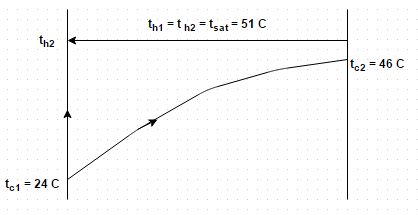# Test: Mean Value Of Capacity Ratio

## 10 Questions MCQ Test Heat Transfer for Engg. | Test: Mean Value Of Capacity Ratio

Description
This mock test of Test: Mean Value Of Capacity Ratio for Chemical Engineering helps you for every Chemical Engineering entrance exam. This contains 10 Multiple Choice Questions for Chemical Engineering Test: Mean Value Of Capacity Ratio (mcq) to study with solutions a complete question bank. The solved questions answers in this Test: Mean Value Of Capacity Ratio quiz give you a good mix of easy questions and tough questions. Chemical Engineering students definitely take this Test: Mean Value Of Capacity Ratio exercise for a better result in the exam. You can find other Test: Mean Value Of Capacity Ratio extra questions, long questions & short questions for Chemical Engineering on EduRev as well by searching above.
QUESTION: 1

### How many fluids remain at constant temperature during the process of boiling and condensation?

Solution:

During the process of boiling and condensation, only a phase change takes place and one fluid remain at constant temperature.

QUESTION: 2

### Identify the correct expression for effectiveness

Solution:

Here, C MAX = infinity and C MIN/C MAX = 0.

QUESTION: 3

### In a gas turbine recuperator, the capacity ratio becomes

Solution:

In a gas turbine recuperator, the exhaust gases after expansion in the turbine are used to heat the compressed air.

QUESTION: 4

In a gas turbine recuperator, the expression for effectiveness for the parallel flow configuration reduces to

Solution:

In a gas turbine recuperator, the exhaust gases after expansion in the turbine are used to heat the compressed air.

QUESTION: 5

What is the maximum efficiency for parallel flow heat exchanger?

Solution:

No matter how large the exchanger be or how high be the flow of overflow at transfer coefficient, the maximum efficiency for parallel flow heat exchanger is 5%.

QUESTION: 6

A two pass surface condenser is required to handle the exhaust from a turbine developing 15 MW with specific steam consumption of 5 kg/k W h. The quality of exhaust steam is 0.9, the condenser vacuum is 66 cm of mercury while the bar meter reads 76 cm of mercury. The condenser tubes are 28 mm inside diameter, 4 mm thick and water flows through tubes with a speed of 3 m/s and inlet temperature 20 degree Celsius. All the steam is condensed, the condensate is saturated water and temperature of cooling water at exit is 5 degree Celsius less than the condensate temperature. Assuming that overall coefficient of heat transfer is 4 k W/m2 degree, determine the mass of cooling water circulatedSolution:

= 76 – 66/76 (1.0133) = 0.133 bar. From energy balance, heat loss by steam = heat gained by cooling water.

QUESTION: 7

The curve of effectiveness versus NTU parameters indicates the relationship between
(i) Effectiveness
(ii) NTU MAX
(iii) C MIN/C MAX
Identify the correct statements

Solution:

When any two of three parameters are known, the third can be find out.

QUESTION: 8

Hot water having specific heat 4200 J/kg K flows through a heat exchanger at the rate of 4 kg/min with an inlet temperature of 100 degree Celsius. A cold fluid having a specific heat 2400 J/kg K flows in at a rate of 8 kg/min and with inlet temperature 20 degree Celsius. Make calculations for maximum possible effectiveness if the fluid flow conforms to parallel flow arrangement

Solution:

Maximum possible effectiveness = 1 – exponential (- infinity)/1 + C = 0.533.

QUESTION: 9

Consider the above problem, make calculations for maximum possible effectiveness if the fluid flow conforms to counter flow arrangement

Solution:

Maximum possible effectiveness = 1 – exponential (- infinity)/ 1 – C exponential (- infinity) = 1.

QUESTION: 10

A counter flow heat exchanger is used to col 2000 kg/hr of oil (cp = 2.5 k J/kg K) from 105 degree Celsius to 30 degree Celsius by the use of water entering at 15 degree Celsius. If the overall heat transfer coefficient is expected to be 1.5 k W/mK, find out the water flow rate. Presume that the exit temperature of the water is not to exceed 80 degree Celsius

Solution:

Mass flow rate of coolant = 2000 (2.5) (105-30)/4.18 (80-15) = 1380.2 kg/hr.